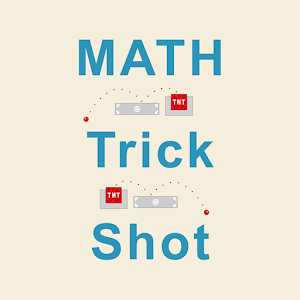English

Every day we offer FREE licensed Android apps and games you’d have to buy otherwise.\$2.99
EXPIRED

# Android Giveaway of the Day - Trick Shot Math

With this premium learning app you can practice math while playing a fun and simple mini-game.
\$2.99 EXPIRED
User rating: 0 (0%) 0 (0%)

This giveaway offer has expired. Trick Shot Math is now available on the regular basis.

With this premium learning app you can practice math while playing a fun and simple mini-game. Trick Shot Math is powered by the most natural handwriting input and has a large selection of math facts from 1st to 6th grade with additional customization options. You can practice the following math skills:

Add a number to a multiple of ten
Add three numbers up to 10 each
Add a two-digit and a one-digit number
Add multiples of 10 or 100
Add two numbers up to three digits
Add three numbers up to two digits each
Add three numbers up to three digits each
Add two numbers with four digits
Complete the addition sentence up to three digits
Balance addition equations up to two digits

Subtraction:
Subtraction facts - numbers up to 10
Subtraction facts - numbers up to 18
Subtract a multiple of ten
Subtraction facts - numbers up to 20
Subtract a one-digit number from a two-digit number
Subtract two two-digit numbers
Subtract multiples of 10 or 100
Balance subtraction equations
Subtraction facts - numbers up to 100
Subtract two three-digit numbers
Complete the subtraction sentence up to three digits
Subtract numbers with four or five digits
Balance subtraction equations up to three digits

Multiplication:
Multiplication tables for 2, 3, 4, 5, 10"
Multiplication tables for 6, 7, 8, 9
Multiply by a multiple of ten
Multiplication facts up to 10×10
Multiplication facts up to 12×12
Multiply one-digit numbers by two-digit numbers
Multiply one-digit numbers by three-digit numbers
Multiply 1-digit numbers by 4-digit numbers
Multiply 2-digit numbers by 2-digit numbers
Multiply numbers ending in zeroes
Multiply three numbers up to 10 each

Division:
Division facts for 2, 3, 4, 5, 10
Division facts for 6, 7, 8, 9
Division facts up to 10
Division facts up to 12
Divide two-digit numbers by one-digit numbers
Divide three-digit numbers by one-digit numbers
Divide three-digit numbers by two-digit numbers
Divide four-digit numbers by one-digit numbers
Divide four-digit numbers by two-digit numbers
Divide numbers ending in zeroes by numbers up to 12

Decimals:
Subtract decimal numbers
Convert decimals to fractions and mixed numbers
Convert fractions and mixed numbers to decimals denominators of 10 and 100
Round decimals to the nearest whole number
Round decimals to the nearest tenth
Round decimals to the nearest hundredth
Multiply a decimal by a power of ten
Multiply a decimal by a one-digit whole number
Multiply two decimal numbers
Divide decimals by powers of ten
Division with decimal quotients
Divide decimals
Convert fractions and mixed numbers to decimals

Fractions:
Subtract fractions with like denominators
Subtract fractions with unlike denominators
Add fractions with denominators of 10 and 100
Multiply fractions by one-digit whole numbers
Multiply fractions by whole numbers
Multiply two fractions
Multiply a mixed number by a fraction
Divide fractions by whole numbers
Divide whole numbers by fractions
Divide two fractions
Write fractions in lowest terms
Add fractions and mixed numbers with like denominators
Add fractions and mixed numbers with unlike denominators
Subtract fractions and mixed numbers with like denominators
Subtract fractions and mixed numbers with unlike denominators
Multiply fractions and mixed numbers
Multiply mixed numbers and whole numbers
Divide fractions and mixed numbers
Divide mixed numbers by whole numbers

Integers:
Subtract integers
Multiply integers
Divide integers
Subtract three integers
Multiply three integers

Sergey Malugin

Educational

### Version:

Varies with device

### Size:

Varies with device

Everyone

### Compatibility:

Varies with device

## Comments on Trick Shot Math

Thank you for voting!